# SPICE it up: How to extract the input capacitance of an op amp (part 3)

Other Parts Discussed in Post: TINA-TI

In operational amplifier (op amp) applications, the feedback resistance of the amplifier interacts with its input capacitance to create a zero in the noise-gain response of the amplifier. This zero in the response, unless properly compensated, reduces the amplifier’s phase margin, causing a peaked frequency response with possible instability – or at the very least ringing in the pulse response.

The amplifier’s input capacitance may be the dominant source capacitance in high-speed transimpedance applications where avalanche photo diodes (APDs) serve as the front-end sensor. An accurate model of the input capacitance is vitally important in such situations to ensure that the simulations closely match bench measurements. Op-amp macro-models with inaccurate input capacitances will result to erroneous simulation results.

In this blog post, I will explore methods to extract the common-mode and differential input capacitance of an amplifier. Figure 1 shows an example of an op amp circuit with the configuration of the internal capacitances explicitly drawn. The differential input capacitance has a minor effect on loop gain, since the amplifier’s closed-loop, virtual-ground behavior bootstraps the input terminals together, thereby lowering the effect of the differential capacitance. The printed circuit board (PCB) parasitic capacitance on the inverting input pin will also affect the loop-gain response and hence its stability. You must take care during layout to minimize this parasitic capacitance.Figure 1: Op-amp model showing input common-mode and differential capacitance

To model the input capacitance of the amplifier, I used an op-amp model without internal input capacitances and added external capacitors. Table 1 lists the amplifier specifications I used.Table 1: Specifications from the example op amp being used

I used different values of common-mode capacitances (inverting and noninverting) for the purposes of illustration. In most op amps, the common-mode capacitance on the inverting and noninverting inputs will be equal; however, current-feedback amplifiers (CFAs) are an exception to this rule.

Figure 2 shows the TINA-TI™ software schematic and Figure 3 shows the simulated closed-loop response of the amplifier.Figure 2: TINA-TI software schematic of an op-amp buffer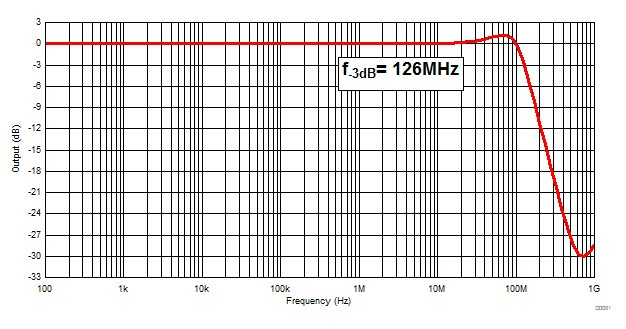Figure 3: Closed-loop bandwidth of a buffer

Noninverting node capacitance (CCM+)

In order to extract CCM+, add a series resistance at the input of the noninverting pin (as shown in Figure 4) and measure the frequency response at the noninverting node. The series resistor forms a low-pass filter (LPF) whose cutoff frequency is a function of the resistor and the internal capacitance. Select a value of resistance such that the -3dB LPF cutoff frequency is much less than the closed-loop bandwidth of the amplifier to ensure that the amplifier is in its linear operating region. You cannot make the value of resistance arbitrarily large. The voltage drop across resistor R1 is due to the bias current and may violate the amplifier’s input common-mode and/or output-swing specifications. Alternatively, you can use an inductor instead of a series resistor to create the LPF. The extracted capacitance, from the simulated frequency response, is:The simulated response of the amplifier measured at its non-inverting input is shown in Figure 5.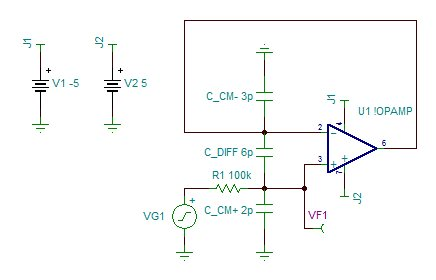Figure 4: TINA-TI software schematic CCM+Figure 5: Simulated response for CCM+ extraction

Inverting node capacitance (CCM-)

In order to extract CCM-, add a feedback resistor (R1) to the buffer configuration. CCM- and R1 create a zero in the noise-gain curve. The zero in the noise-gain curve peaks the amplifier’s frequency response. Choose the value of R1 carefully so that the location of the zero is much lower than the closed-loop gain of the amplifier to prevent its open-loop gain limitations from affecting your calculations. Figures 6 and 7 show the circuit for this test and the results, respectively. The extracted capacitance, from the simulated frequency response, is: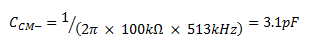Figure 6: TINA-TI software schematic CCM-Figure 7: Simulated response for CCM- extraction

Differential capacitance (CDIFF)

Measuring CDIFF is a little more complicated, as the virtual ground of the amplifier forces its input nodes to track each other – thus bootstrapping the capacitor’s terminals. Figure 8 shows the circuit used to measure CDIFF. The amplifier is in an inverting configuration at DC; however, the feedback inductor puts the amplifier in an open-loop configuration at higher frequencies. The input resistor R1 and the parallel combination of CCM- and CDIFF form a low-pass filter, whose corner frequency is measured by probing the amplifier’s inverting terminal. The extracted capacitance, from the simulated frequency response, is thus: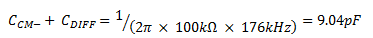The simulated frequency response of the circuit in Figure 8 is shown in Figure 9.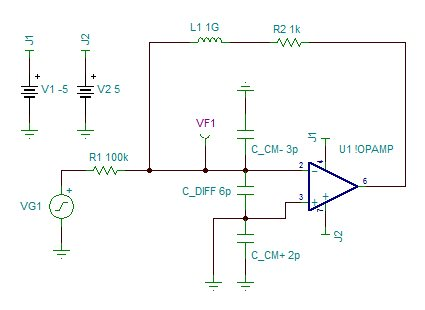Figure 8: TINA-TI software schematic CDIFFFigure 9: Simulated response for CDIFF extraction

In cases where the capacitance modeled is larger than the specified data sheet value, TINA-TI software allows the use of a negative capacitor to correct the modeled value.

I have covered some of the methods for extracting the common-mode and differential input capacitance of an amplifier; however, these are not the only ways. In what ways have you extracted an amplifier’s common-mode and differential input capacitance? Please log in to your TI E2E™ Community account and leave a comment below so we can start a discussion.

The amplifier will be unstable however that will not affect the "simulated" measurement since it occurs at a much lower frequency than the loop gain crossover. Below is my stability analysis:

Aol pole at 1kHz implies a 90 degree phase shift in the loop gain by 10 kHz.

Noise Gain zero at 500 kHz (approx.) implies a 90 degree phase shift by 5 MHz.

The loop gain crossover will occur at the geometric mean of 500kHz and 100MHz which is around 7 MHz. Since this is greater than the 5 MHz a total phase shift of 180degrees has occurred =>unstable.

-Samir

• Thank you for the information.

For the ckt in Fig.6, Noise gain has one zero and rate of closure is 40 dB/decade . Could you comment on the stability of the system.

• Thanks Neil,

I understand the frustrations of working with incomplete models since I face them myself when trying to optimize my designs. We are currently working on improving many of the older models where we have found errors and I am optimistic that they will be fixed this year.

• Nice article, Shamir.

If TI published accurate op amp models this would not be necessary. Some TI macromodels do not include input capacitance- this is unacceptable.